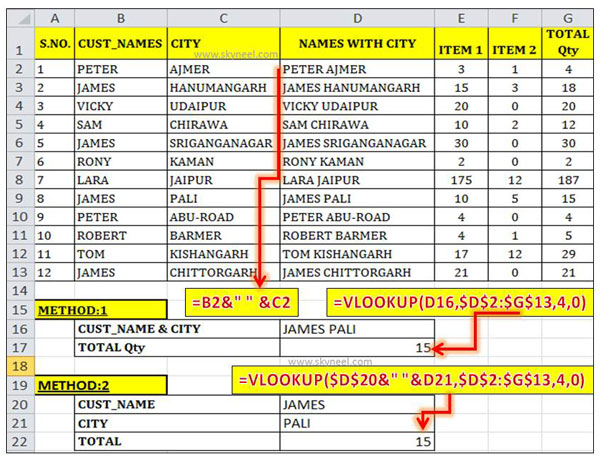# How to use VLookup function with multiple criteria

This article helps you to apply Vlookup function with multiple criteria to search any specific value. Vlookup does not support multiple criteria.

Hi Guys, today we are talking on VLookup function with multiple criteria and make easy to find any specific data as per your need. there are lot of built-in functions and formulas in MS Excel to search and analyse the data. If you are working with a large worksheet or database and want to find any specific data as per your need it will be difficult. There are lot of functions which helps you to quickly find the specific data and provide the exact results in front of you.

One of the most important function is used for searching the specific data is VLookup. All we know very well about the VLookup that it is a great function which is used for searching the value. But you will face the problem when you have more than one criteria because VLookup does not support multiple conditions. So, this article will helps you how to use VLookup function with multiple criteria.

### How to use VLookup function with multiple criteria

If there are multiple records in your active worksheet or database which have same information, then VLookup provide the first one as the result. If you need to apply more than one criteria to match any specific data then you will not able to get the exact result. Most of the users face this kind of situation during searching any specific value using VLookup with multiple criteria. Let’s have discuss in brief how to solve this problem but before it first we know about the VLookup function.

#### Definition & Syntax of VLookup Function:

VLookup is a great function which is found in the “Lookup and Reference” categories in MS Excel. It is used for lookup a value in the left most column of a table, and then return a value in the same row from a column you specify. The VLookup function searches a value in the extreme left column of the table_array and provides the value in the same row which is based on the index number.

= VLookup (lookup_value, table_array, col_index_num, [range])

Note: Before using the VLookup function, first you need to arrange first column of a table or array in the ascending order, otherwise VLookup function is not able to provide the accurate result.

Lookup_value: The value to be searched in the extreme first column of the table array.

Table_array: Two or more columns of data. Give cell reference of a range / range name.

Col_index_num: The column index_number in the table from which the searched value must be returned.

If col_index_num of returns 1 the value in the first column in table_array.

If col_index_num is: Less than 1, this function returns an error value the #VALUE!.

If it is a greater than the number of columns in table_array, this function returns error value #REF!.

Range_lookup: It is an options part of this function. There are two options available in this part.

TRUE: Appropriate Match: This option work properly when first column of your table will be sorted in ascending order only otherwise it displays wrong
result.

False: Exact Match: VLookup by default check only on exact match method.

For E.g.: If you have number of records in a database or worksheet. There are lot or records have same name but other things will be different. Now you want to search any specific values which matched the name and city in the worksheet. In that situation you must have to use VLookup function with multiple criteria.

#### Method 1: VLookup function with multiple criteria

In this method you have to use ampersand (&) operator to combine customer name and city in a new column. After that you will be able to search the specific value which have multiple criteria using VLookup function.

Step 1: Create the following table in any worksheet to apply VLookup function with multiple criteria.Step 2: If you want to search total qty which matched with the cust name “James” who lives in the “Pali” city. You must have to use ampersand (&) operator to combine both cust_name and city columns. You have to insert a new column after the City which have both Cust_name & city. Now apply the formula in cell D2: =B2&” ” &C2. Drag this formula for the rest of cells to get the combination of cust_name and city both.

Step 3: Apply the following VLookup function in cell D17 =VLOOKUP(D16,\$D\$2:\$G\$13,4,0). After pressing the enter key you will get the result 15.

#### Method 2: VLookup function with multiple criteria

In this method there is no need to create another column to apply VLookup function with multiple criteria. You must apply ampersand (&) operator during typing the formula in any cell.

Step 1: Create the following table to start lookup process.

Step 2: Take the cursor on cell D22 and type the following given formula to get the exact result:
=VLOOKUP(\$D\$20&” “&D21,\$D\$2:\$G\$13,4,0).

Step 3: After pressing the enter key you will get the same result 15.

I hope you like this article. After reading this article you will be able to easily apply VLookup function with multiple criteria. Give your important suggestion in the comment box regarding this article. Thanks to all.